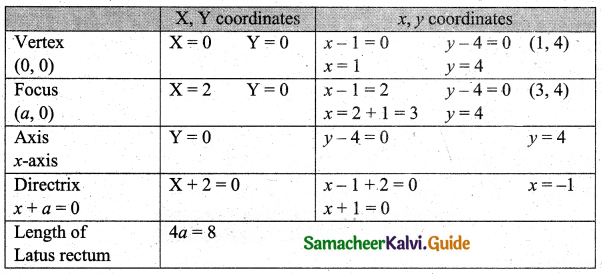Students can download 11th Business Maths Chapter 3 Analytical Geometry Ex 3.6 Questions and Answers, Notes, Samcheer Kalvi 11th Business Maths Guide Pdf helps you to revise the complete Tamilnadu State Board New Syllabus, helps students complete homework assignments and to score high marks in board exams.

## Tamilnadu Samacheer Kalvi 11th Business Maths Solutions Chapter 3 Analytical Geometry Ex 3.6

### Samacheer Kalvi 11th Business Maths Analytical Geometry Ex 3.6 Text Book Back Questions and Answers

Question 1.
Find the equation of the parabola whose focus is the point F(-1, -2) and the directrix is the line 4x – 3y + 2 = 0.
Solution:
F(-1, -2)
l : 4x – 3y + 2 = 0
Let P(x, y) be any point on the parabola.
FP = PM
⇒ FP2 = PM2
⇒ (x + 1)2 + (y + 2)2 = $$\left[\frac{4 x-3 y+2}{\sqrt{4^{2}+(-3)^{2}}}\right]^{2}$$
⇒ x2 + 2x + 1 + y2 + 4y + 4 = $$\frac{16 x^{2}+9 y^{2}+4-24 x y+16 x-12 y}{(16+9)}$$
⇒ 25(x2 + y2 + 2x + 4y + 5) = 16x2 + 9y2 – 24xy + 16x – 12y + 4
⇒ (25 – 16)x2 + (25 – 9)y2 + 24xy + (50 – 16)x + (100 + 12)y + 125 – 4 = 0
⇒ 9x2 + 16y2 + 24xy + 34x + 112y + 121 = 0Question 2.
The parabola y2 = kx passes through the point (4, -2). Find its latus rectum and focus.
Solution:
y2 = kx passes through (4, -2)
(-2)2 = k(4)
⇒ 4 = 4k
⇒ k = 1
y2 = x = 4($$\frac{1}{4}$$)x
a = $$\frac{1}{4}$$
Equation of LR is x = a or x – a = 0
i.e., x = $$\frac{1}{4}$$
⇒ 4x = 1
⇒ 4x – 1 = 0
Focus (a, 0) = ($$\frac{1}{4}$$, 0)

Question 3.
Find the vertex, focus, axis, directrix, and the length of the latus rectum of the parabola y2 – 8y – 8x + 24 = 0.
Solution:
y2 – 8y – 8x + 24 = 0
⇒ y2 – 8y – 42 = 8x – 24 + 42
⇒ (y – 4)2 = 8x – 8
⇒ (y – 4)2 = 8(x – 1)
⇒ (y – 4)2 = 4(2) (x – 1)
∴ a = 2
Y2 = 4(2)X where X = x – 1 and Y = y – 4Question 4.
Find the co-ordinates of the focus, vertex, equation of the directrix, axis and the length of latus rectum of the parabola (a) y2 = 20x, (b) x2 = 8y, (c) x2 = -16y
Solution:
(a) y2 = 20x
y2 = 4(5)x
∴ a = 5(b) x2 = 8y = 4(2)y
∴ a = 2(c) x2 = -16y = -4(4)y
∴ a = 4Question 5.
The average variable cost of the monthly output of x tonnes of a firm producing a valuable metal is ₹ $$\frac{1}{5}$$ x2 – 6x + 100. Show that the average variable cost curve is a parabola. Also, find the output and the average cost at the vertex of the parabola.
Solution:
Let output be x and average variable cost = y
y = $$\frac{1}{5}$$ x2 – 6x + 100
⇒ 5y = x2 – 30x + 500
⇒ x2 – 30x + 225 = 5y – 500 + 225
⇒ (x – 15)2 = 5y – 275
⇒ (x – 15)2 = 5(y – 55) which is of the form X2 = 4($$\frac{5}{4}$$)Y
∴ Y average variable cost curve is a parabola
Vertex (0, 0)
x – 15 = 0; y – 55 = 0
x = 15; y = 55
At the vertex, output is 15 tonnes and average cost is ₹ 55.Question 6.
The profit ₹ y accumulated in thousand in x months is given by y = -x2 + 10x – 15. Find the best time to end the project.
Solution:
y = -x2 + 10x – 15
⇒ y = -[x2 – 10x + 52 – 52 + 15]
⇒ y = -[(x – 5)2 – 10]
⇒ y = 10 – (x – 5)2
⇒ (x – 5)2 = -(y – 10)
This is a parabola which is open downwards.
Vertex is the maximum point.
∴ Profit is maximum when x – 5 = 0 (or) x = 5 months.